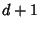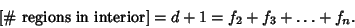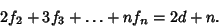## Grinberg Formula

A formula satisfied by all Hamiltonian Circuits withnodes. Letbe the number of regions inside the circuit withsides, and letbe the number of regions outside the circuit withsides. If there areinterior diagonals, then there must beregions(1)

Any region withsides is bounded byEdges, so such regions contributeto the total. However, this counts each diagonal twice (and each Edge only once). Therefore,(2)

Take (2) minus(1),(3)

Similarly,(4)

so(5)